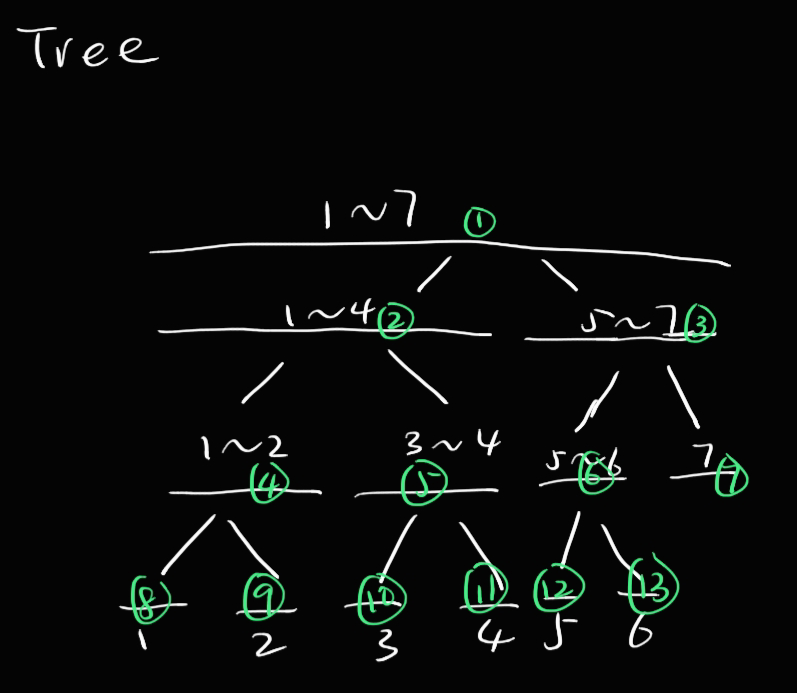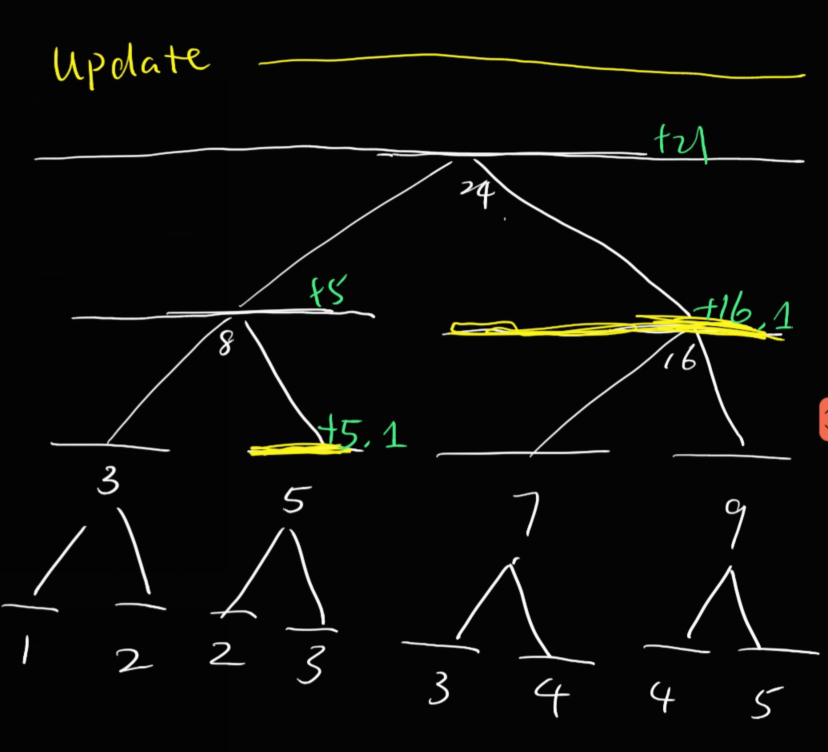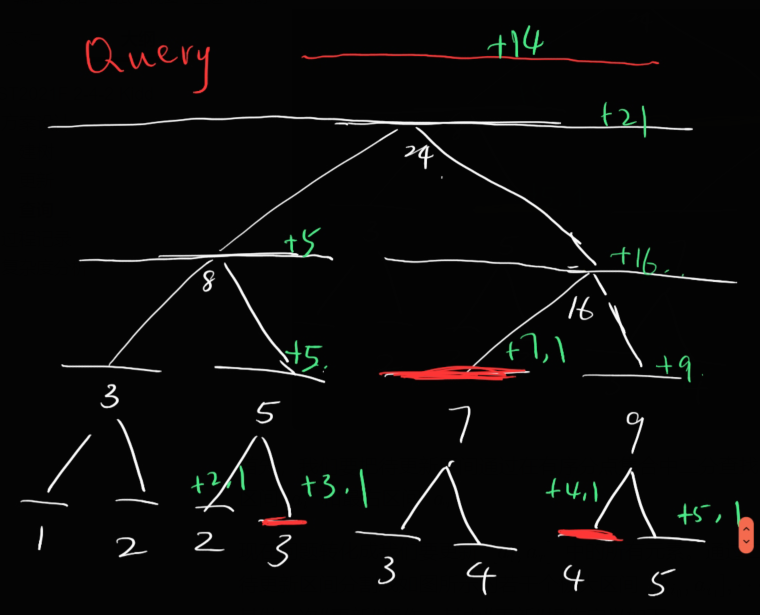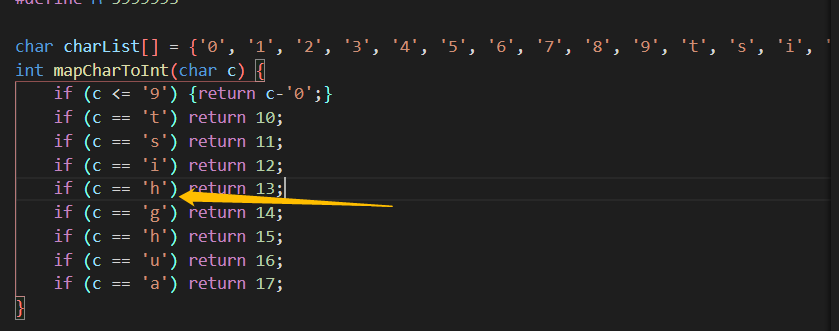• CST2021F 2-1 Build
• CST2021F 2-4-2 Kidd
• CST2021F 2-6 Hacker

Problem White Box (20%) Black Box (80%)
CST2021F 2-1 Build (25%) 100  -1
CST2021F 2-4-2 Kidd (25%) 95  -1
CST2021F 2-6 Hacker (25%) 100  -2

## CST2021F 2-1 NotFound

[关键词] 父亲-长子-兄弟法的多叉树表示，List

### 方案设计

#### 结点

• parent，指向父亲的指针
• firstChild，指向长子的指针
• nextSibling，指向下一个兄弟的指针
• prevSibling，指向前一个兄弟的指针
• height，子树高度
• size，子树规模
• suffixMaxHeight，以自己为基点，自己及自己之后的兄弟的高度的最大值
• childLength，孩子个数
• childPushed，在建树时用到的临时变量

#### 建树

• 如果一个结点的 childPushedfalse，那么将其该字段设为 true，并将其压入栈中，将其所有孩子按照长子到末子的顺序压入栈中。
• 如果一个结点是叶节点，那么直接将其预处理。
• $height \leftarrow 0$​
• $size \leftarrow 1$
• $suffixMaxHeight \leftarrow \max(suffixMaxHeight_{nextSibling?}, height)$​​
• $parent?.height \leftarrow parent?.height+1$
• 不然我们得出该结点一定有长子，且其所有孩子一定先于其被预处理。
• $height \leftarrow firstChild.suffixMaxHeight +1$
• $size \leftarrow size + 1$​
• $suffixMaxHeight \leftarrow \max(suffixMaxHeight_{nextSibling?}, height)$
• $parent?.height \leftarrow parent?.height+1$
• 其中 $?$ 表示对其不存在的情况做特判。

#### 删除子树

• 改变子树局部的拓扑结构
• 沿“祖父”链更新子树规模
• 沿“结点$\rightarrow$长子$\rightarrow$父亲$\rightarrow\cdots$”链更新 heightsuffixMaxHeight

#### 插入子树

• 改变子树局部的拓扑结构
• 沿“祖父”链更新子树规模
• 沿“结点$\rightarrow$长子$\rightarrow$父亲$\rightarrow\cdots$”链更新 heightsuffixMaxHeight

## CST2021F 2-2 NotFound

[AnsLen = 1]

1 01 1
0 11 2

[AnsLen = 2]

10 0010 1
01 0110 2
10 0110 2
00 1110 3
00 1110 3
01 1110 3
11 1111 4

[AnsLen = 3]

101 00000100 1
010 00100100 2
100 00101100 3
000 10101100 4
001 11101100 5
011 11111100 6

### 复杂度分析

# Judger by c7w
import io
import time
import datetime
import random
import subprocess
import numpy as np

class Judger:
def generateInputData(self):
IN = ''
for i in range(2**24):
IN += f'{random.randint(0, 1)}'
IN += '\n'
return IN

OUT = ''
return OUT.strip()

def judge(self, name, judgeAns=True):
print('\n\nCompiling...')
try:
subprocess.check_output(['g++', f'{name}', '-o', 'main'])
except subprocess.CalledProcessError as e:
return 'CE'

print('Generating testing data...')
data = self.generateInputData()

print('Running...')
try:
time1 = time.time()
cppout = subprocess.check_output(['./main'], input=bytes(data, 'utf-8'), timeout=2.0)
cppout = cppout.decode('utf-8').strip()
time2 = time.time()
except subprocess.TimeoutExpired:
f = open(f'check-{datetime.datetime.now().strftime("%Y-%m-%d-%H-%M-%S")}.log', 'w+', encoding='utf-8')
f.write(f"Time Limit Exceeded\n")
f.write("-------------IN--------------\n")
f.write(data + '\n')
f.close()
return f'Time Limit Exceeded (2.000s)'
except subprocess.CalledProcessError as e:
f = open(f'check-{datetime.datetime.now().strftime("%Y-%m-%d-%H-%M-%S")}.log', 'w+', encoding='utf-8')
f.write(f"Runtime Error (signal {e.returncode})\n")
f.write("-------------IN--------------\n")
f.write(data + '\n')
f.close()
return f'Runtime Error (Signal {e.returncode})'

if judgeAns:
# print(cppout)
# print("↑ cppout ↓ ans")
# print(ans)

print('Judging...')
try:
cppout_ = cppout.split('\n')
ans_ = ans.split('\n')
if (len(cppout_) != len(ans_)):
raise BaseException(-1)
for i in range(len(cppout_)):
if (cppout_[i].strip() != ans_[i].strip()):
raise BaseException(i)
except BaseException as e:
f = open(f'check-{datetime.datetime.now().strftime("%Y-%m-%d-%H-%M-%S")}.log', 'w+', encoding='utf-8')
f.write("-------------IN--------------\n")
f.write(data + '\n')
f.write("-------------CPPOUT--------------\n")
f.write(cppout + '\n')
f.write("-------------ANS--------------\n")
f.write(ans + '\n')
f.close()
return f'Accepted ({round(time2 - time1, 3)}s)'

else: # Do not judge answer
return f'Program exited ({round(time2 - time1, 3)}s)'

j = Judger()
for i in range(10):
print(j.judge('main.cpp', False))

## CST2021F 2-4-2 Kidd

[关键词] Segment Tree

### 方案设计

#### 建树#### 更新• 将自身和自身的所有祖先的 $sum$ 加上自己的权值，表示对应区间翻转次数增加这个权值。
• 将自身的“懒惰”标记 $lazy$ 增加 1，表示有一次翻转并没有通知自己的子节点。

#### 查询• 在“分割”成极大子区间的过程中，如果一个含有懒惰标记的区间 $a_i$​​ 被分割，那么将该懒惰标记释放，同时其两个子区间 $a_j, a_k$​​ 要更新其 $sum$​​ 值和 $lazy$ 值：
• $sum_j \leftarrow sum_j + weight_j*lazy_i$​
• $sum_k \leftarrow sum_k + weight_k*lazy_i$​
• $lazy_j \leftarrow lazy_j + lazy_i$
• $lazy_k \leftarrow lazy_k + lazy_i$
• 事实上，这是对之前更新时并未更新子区间的翻转次数和的“延时效应”的激活。
• 返回各个极大子区间的 $sum$ 值之和。

## CST2021F 2-6 Hacker

### 方案设计

#### 密码表示

• ‘0’ ~ ‘9’ 映射至 0 ~ 9
• ‘t’, ‘s’, ‘i’, ‘n’, ‘g’, ‘h’, ‘u’, ‘a’ 映射至 10 ~ 17

• 使用 long long ($64$ Bit) 来表示一个密码
• 密码的后 $4$ 个 Bit 表示密码长度.
• 密码的后 $[5k+4, 5k+9)$​ 个 Bit 表示倒数第 $k$ 个字符。

### 过程记录《当你看代码逻辑三小时而不知出什么问题.jpeg》
• 初版代码忘了在历史记录插入 HashTable 时加盐（样例都跑不过 本地解决）
• 第一次九成测内存空间没算对。密码结点最多数目为 $2M$（Init）+ $3M$​（历史记录插入）